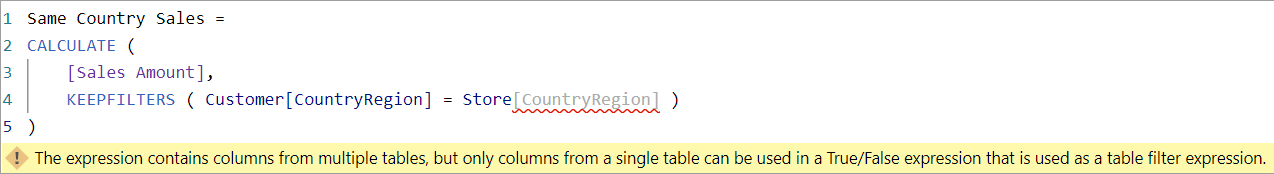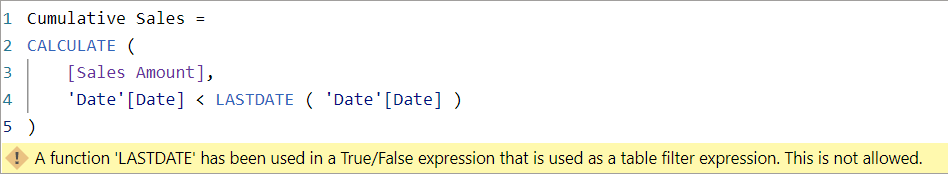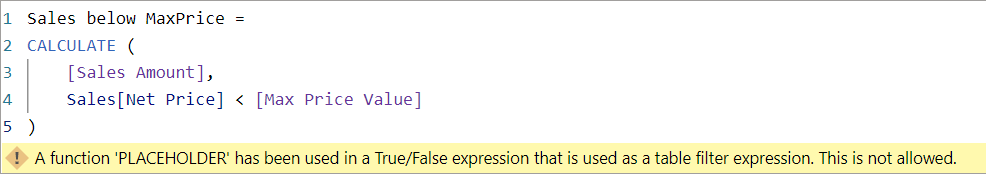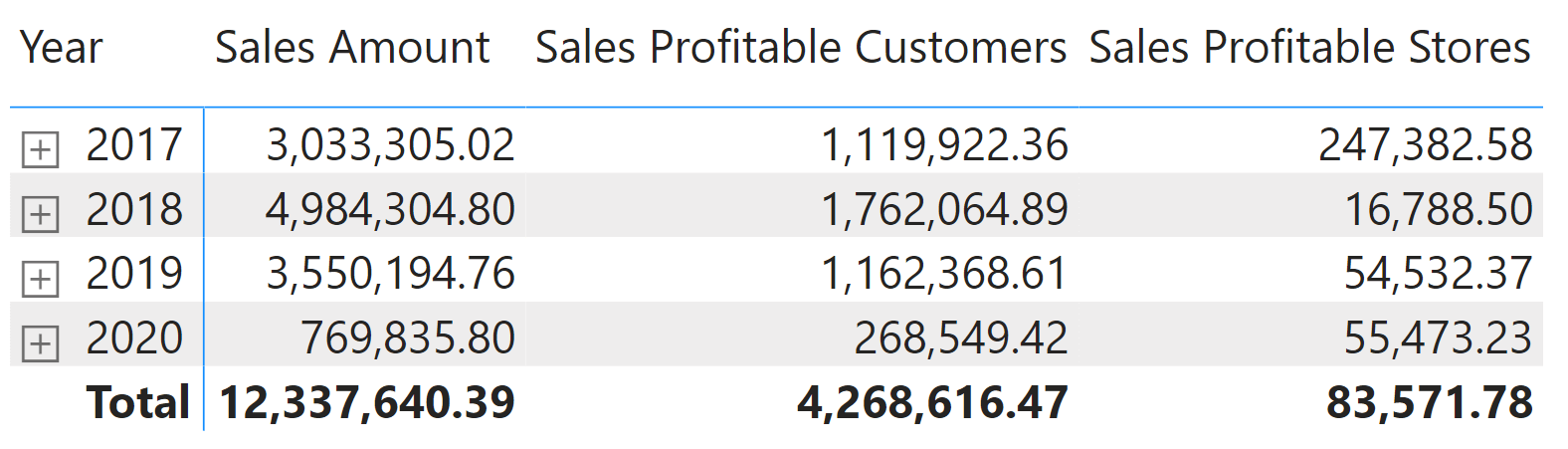When you write a filter argument in CALCULATE, you provide a table that is placed in the filter context. However, CALCULATE provides a simplified syntax where the table is automatically created based on a filter condition (predicate). The filter condition can include one or more column references from the same table: each filter argument is converted into a corresponding table expression that returns the table to place in the filter context. For example, consider the following CALCULATE expression:

```CALCULATE (
[Sales Amount],
KEEPFILTERS ( Sales[Quantity] * Sales[Net Price] > 1000 ),
Customer[Country] IN { "Canada", "United States" },
'Product'[Brand] = "Contoso"
)
```

The corresponding syntax with the table expression generated by the filter predicates is as follows:

```CALCULATE (
[Sales Amount],
KEEPFILTERS (
FILTER (
ALL ( Sales[Quantity], Sales[Net Price] ),
Sales[Quantity] * Sales[Net Price] > 1000
)
),
FILTER (
ALL ( Customer[Country] ),
Customer[Country] IN { "Canada", "United States" }
),
FILTER (
ALL ( 'Product'[Brand] ),
'Product'[Brand] = "Contoso"
)
)
```

The shorter syntax is easier to read, but it is important to remember that every condition must be translated into a corresponding FILTER table expression. When this translation is impossible, the result is a syntax error with an error message that is not always intuitive.

## Common errors

Let us examine the more common error messages obtained when an invalid predicate is in a filter argument of CALCULATE or CALCULATETABLE.

### The expression contains columns from multiple tables, but only columns from a single table can be used in a True/False expression that is used as a table filter expression.

This error is seen when the predicate includes column references from more than one table. For example, if we need a measure that returns the sales made to customers living in the same country as the store, we could try to write the following measure:

Measure in Sales table
```Same Country Sales :=
CALCULATE (
[Sales Amount],
KEEPFILTERS ( Customer[CountryRegion] = Store[CountryRegion] )
)
```

The result is the error message, “The expression contains columns from multiple tables, but only columns from a single table can be used in a True/False expression that is used as a table filter expression.”Because DAX can only translate the filter condition in a FILTER over an ALL function, the columns must be from the same table. We must create a table for FILTER by getting columns from different tables using CROSSJOIN or SUMMARIZE. In this case, because the cardinality of the columns involved is small, CROSSJOIN is the best approach:

Measure in Sales table
```Same Country Sales :=
CALCULATE (
[Sales Amount],
KEEPFILTERS (
FILTER (
CROSSJOIN ( ALL ( Customer[CountryRegion] ), ALL ( Store[CountryRegion] ) ),
Customer[CountryRegion] = Store[CountryRegion]
)
)
)
```

You can also try the code with the following DAX query:

```DEFINE MEASURE Sales[Same Country Sales] =
CALCULATE (
[Sales Amount],

-- Invalid condition
-- KEEPFILTERS ( Customer[CountryRegion] = Store[CountryRegion] )

-- Valid condition
KEEPFILTERS (
FILTER (
CROSSJOIN ( ALL ( Customer[CountryRegion] ), ALL ( Store[CountryRegion] ) ),
Customer[CountryRegion] = Store[CountryRegion]
)
)
)
EVALUATE
SUMMARIZECOLUMNS (
'Product'[Brand],
"Sales Amount", [Sales Amount],
"Same Country Sales", [Same Country Sales]
)
```
Product[Brand] Sales Amount Same Country Sales
Contoso 7,352,399.03 2,477,499.14
Wide World Importers 1,901,956.66 662,887.57
Southridge Video 1,384,413.85 533,991.83
Litware 3,255,704.03 1,285,931.51
Fabrikam 5,554,015.73 2,053,525.97
Proseware 2,546,144.16 959,471.95
A. Datum 2,096,184.64 769,212.16
The Phone Company 1,123,819.07 356,847.25
Tailspin Toys 325,042.42 100,018.04

### A function ‘CALCULATE‘ has been used in a True/False expression that is used as a table filter expression. This is not allowed.

When the filter argument is expressed as a filter condition, the presence of a function that performs a context transition is not allowed. For this reason, you cannot use CALCULATE in the predicate of a CALCULATE or CALCULATETABLE call.

Consider the requirement for a measure to return the sales amount of products with a high price, where the boundary is 90% of the highest price available. We could try the following implementation:

Measure in Sales table
```Sales Top Prices :=
CALCULATE (
[Sales Amount],
Sales[Net Price] > CALCULATE ( MAX ( Sales[Net Price] ) * 0.9, REMOVEFILTERS() )
)
```

However, this definition returns the error, “A function ‘CALCULATE‘ has been used in a True/False expression that is used as a table filter expression. This is not allowed.”The presence of a context transition in the predicate is the reason why the syntax is not translated into an equivalent FILTER.In this case, it is possible to create an explicit FILTER without modifying the original filter condition:

Measure in Sales table
```Sales Top Prices :=
CALCULATE (
[Sales Amount],
FILTER (
ALL ( Sales[Net Price] ),
Sales[Net Price] > CALCULATE ( MAX ( Sales[Net Price] ) * 0.9, REMOVEFILTERS() )
)
)
```

In general, this is not a good idea. In this specific example, we can do that because CALCULATE also includes a REMOVEFILTER, which removes the effect of the context transition. This way, the presence of a context transition in the FILTER iterator does not have undesired side effects. But most of the time, CALCULATE would not remove the filter context, which is usually an undesired side effect. For these reasons, a better approach is to move the CALCULATE function used in the predicate outside the filter argument, and store the result in a variable used in the filter argument:

Measure in Sales table
```Sales Top Prices - best practice :=
VAR NetPriceLimit =
CALCULATE (
MAX ( Sales[Net Price] ) * 0.9,
REMOVEFILTERS()
)
VAR Result =
CALCULATE (
[Sales Amount],
Sales[Net Price] > NetPriceLimit
)
RETURN Result
```

You can test the two measures by trying the following DAX query:

```DEFINE
MEASURE Sales[Sales Top Prices] =
CALCULATE (
[Sales Amount],
FILTER (
ALL ( Sales[Net Price] ),
Sales[Net Price] > CALCULATE ( MAX ( Sales[Net Price] ) * 0.9, REMOVEFILTERS() )
)
)

MEASURE Sales[Sales Top Prices - best practice] =
VAR NetPriceLimit =
CALCULATE ( MAX ( Sales[Net Price] ) * 0.9, REMOVEFILTERS() )
VAR Result =
CALCULATE (
[Sales Amount],
Sales[Net Price] > NetPriceLimit
)
RETURN Result

EVALUATE
SUMMARIZECOLUMNS (
'Product'[Brand],
"Sales Amount", [Sales Amount],
"Sales Top Prices", [Sales Top Prices],
"Sales Top Prices - best practice", [Sales Top Prices - best practice]
)

```
Product[Brand] Sales Amount Sales Top Prices Sales Top Prices – best practice
Contoso 7,352,399.03 (Blank) (Blank)
Wide World Importers 1,901,956.66 (Blank) (Blank)
Southridge
Video
1,384,413.85 (Blank) (Blank)
Litware 3,255,704.03 220,799.31 220,799.31
Fabrikam 5,554,015.73 179,199.44 179,199.44
Proseware 2,546,144.16 (Blank) (Blank)
A. Datum 2,096,184.64 (Blank) (Blank)
The Phone Company 1,123,819.07 (Blank) (Blank)
Tailspin Toys 325,042.42 (Blank) (Blank)

The CALCULATE function mentioned in the error message could be replaced by any other function that performs a context transition. For example, consider the following measure:

Measure in Sales table
```Cumulative Sales :=
CALCULATE (
[Sales Amount],
'Date'[Date] <= LASTDATE ( 'Date'[Date] )
)
```

In this case the error message is, “A function ‘LASTDATE‘ has been used in a True/False expression that is used as a table filter expression. This is not allowed.”This is a case where manually writing the FILTER over ALL would not produce the expected result, because LASTDATE would produce a different result for each iterated row from Date[Date]. Moreover, it does not make sense to use LASTDATE when a scalar value is required. LASTDATE should be used only as a table function. Therefore, the first solution could be to use MAX instead of LASTDATE:

Measure in Sales table
```Cumulative Sales :=
CALCULATE (
[Sales Amount],
'Date'[Date] <= MAX ( 'Date'[Date] )
)
```

However, it is always a best practice to move aggregations into variables to get the desired value and reduce the number of evaluations requested:

Measure in Sales table
```Cumulative Sales - best practice :=
VAR LastVisibleDate = MAX ( 'Date'[Date] )
VAR Result =
CALCULATE (
[Sales Amount],
'Date'[Date] <= LastVisibleDate
)
RETURN Result
```

You can test the two measures by trying the following DAX query:

```DEFINE
MEASURE Sales[Cumulative Sales] =
CALCULATE (
[Sales Amount],
'Date'[Date] <= MAX ( 'Date'[Date] )
)

MEASURE Sales[Cumulative Sales - best practice] =
VAR LastVisibleDate = MAX ( 'Date'[Date] )
VAR Result =
CALCULATE (
[Sales Amount],
'Date'[Date] <= LastVisibleDate
)
RETURN Result

EVALUATE
SUMMARIZECOLUMNS (
'Date'[Calendar Year],
"Sales Amount", [Sales Amount],
"Cumulative Sales", [Cumulative Sales],
"Cumulative Sales - best practice", [Cumulative Sales - best practice]
)
ORDER BY 'Date'[Calendar Year]
```
Date[Calendar Year] Sales Amount Cumulative Sales Cumulative Sales – best practice
CY 2007 11,309,946.12 11,309,946.12 11,309,946.12
CY 2008 9,927,582.99 21,237,529.11 21,237,529.11
CY 2009 9,353,814.87 30,591,343.98 30,591,343.98
CY 2010 (Blank) 30,591,343.98 30,591,343.98
CY 2011 (Blank) 30,591,343.98 30,591,343.98

### A function ‘PLACEHOLDER’ has been used in a True/False expression that is used as a table filter expression. This is not allowed.

As previously described, we always have the same error message when there is a function that performs a context transition in the syntax of a predicate filter argument: the only difference is the offending function name cited in the error message. However, DAX does not have a PLACEHOLDER function, but there is a specific scenario where this is the error message displayed for a filter argument of CALCULATE: when you use a measure reference in the predicate.

We are going to see two common occurrences of this issue. The first is a measure reference to read a slicer value. For example, the following report shows for each year the overall Sales Amount and the Sales Amount of transactions with a Net Price less than the value selected in the slider.The Max Price slider is built using the Power BI numeric range parameter. Power BI created a Max Price Value measure in the model that returns the selection made in the Max Price slider. We try to write the condition comparing the Sales[Net Price] column with the Max Price Value measure:

Measure in Sales table
```Sales below MaxPrice :=
CALCULATE (
[Sales Amount],
Sales[Net Price] < [Max Price Value]
)
```

This code just generates an error, this time using the name PLACEHOLDER instead of the offending function name. We get, “A function ‘PLACEHOLDER’ has been used in a True/False expression that is used as a table filter expression. This is not allowed.”Instead of PLACEHOLDER, the right name to use should be CALCULATE. Indeed, every time there is a measure reference, it could be replaced by CALCULATE ( <measure definition> ). However, the error message leaves the generic PLACEHOLDER name instead of mentioning CALCULATE. Nevertheless, the issue is always the same: the presence of a context transition in a predicate filter argument. Thus, the solution is still the same. We should evaluate the measure reference and assign the result to a variable before CALCULATE:

Measure in Sales table
```Sales below MaxPrice :=
VAR MaxPrice = [Max Price Value]
VAR Result =
CALCULATE (
[Sales Amount],
Sales[Net Price] < MaxPrice
)
RETURN Result
```

The second occurrence of this issue is when a measure reference without any column reference is used. For example, the following Sales Profitable measure should display the sales with a margin greater than 60%:

Measure in Sales table
```Sales Profitable :=
CALCULATE (
[Sales Amount],
[Margin %] > 0.6
)
```

The result is always the same error, “A function ‘PLACEHOLDER’ has been used in a True/False expression that is used as a table filter expression. This is not allowed.”In this case, evaluating the measure reference outside of CALCULATE would not be a solution because the real issue is that we do not have a definition of the target filter. In other words, the requirement is incomplete. Should we filter the sales where the margin for a customer is greater than 60%? Or is it the margin for a store that should be greater than 60%? To write the DAX measure, it is necessary to clarify the requirements beforehand.

For example, if the requirement is to show Sales Amount for the customers that have a margin greater than 60%, then this is the correct measure:

Measure in Sales table
```Sales Profitable Customers :=
CALCULATE (
[Sales Amount],
FILTER (
Customer,
[Margin %] > 0.6
)
)
```

Otherwise, if the requirement is to show Sales Amount for the stores with a margin greater than 60%, then we should write the following measure:

Measure in Sales table
```Sales Profitable Stores :=
CALCULATE (
[Sales Amount],
FILTER (
Store,
[Margin %] > 0.6
)
)
```

The Sales Profitable Customers and Sales Profitable Stores measures return different numbers.This was to be expected because the filters are applied to different business entities (Customer and Store). Thus, when the predicate includes the target of a filter, the measure reference can be evaluated and assigned to a variable before CALCULATE. When the predicate has a measure reference and does not specify a filter target, then the measure reference should be evaluated in a row context e the target of the filter.

## Conclusions

The filter arguments in CALCULATE can be written using a predicate, which makes the code easier to read and maintain. The predicate has a few limitations related to the columns that can be included – which must be part of the same table – and the presence of functions that perform a context transition, including any measure reference. The solution is usually to move the calculation outside the predicate, most of the time into a variable evaluated just before CALCULATE. When a predicate has only a measure reference without any table/column reference, then you must figure out the filter target and evaluate the measure reference while iterating the business entity to filter.

CALCULATEContext transition

Evaluates an expression in a context modified by filters.

`CALCULATE ( <Expression> [, <Filter> [, <Filter> [, … ] ] ] )`

FILTERReturns a table that has been filtered.

`FILTER ( <Table>, <FilterExpression> )`

CALCULATETABLEContext transition

Evaluates a table expression in a context modified by filters.

`CALCULATETABLE ( <Table> [, <Filter> [, <Filter> [, … ] ] ] )`

ALLCALCULATE modifier

Returns all the rows in a table, or all the values in a column, ignoring any filters that might have been applied.

`ALL ( [<TableNameOrColumnName>] [, <ColumnName> [, <ColumnName> [, … ] ] ] )`

CROSSJOINReturns a table that is a crossjoin of the specified tables.

`CROSSJOIN ( <Table> [, <Table> [, … ] ] )`

SUMMARIZECreates a summary of the input table grouped by the specified columns.

`SUMMARIZE ( <Table> [, <GroupBy_ColumnName> [, [<Name>] [, [<Expression>] [, <GroupBy_ColumnName> [, [<Name>] [, [<Expression>] [, … ] ] ] ] ] ] ] )`

LASTDATEContext transition

Returns last non blank date.

`LASTDATE ( <Dates> )`

MAXReturns the largest value in a column, or the larger value between two scalar expressions. Ignores logical values. Strings are compared according to alphabetical order.

`MAX ( <ColumnNameOrScalar1> [, <Scalar2>] )`